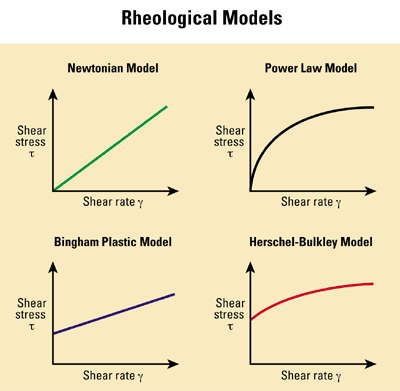# Herschel–Bulkley fluid

1. . []

A fluid described by a three-parameter rheological model. A Herschel–Bulkley fluid can be described mathematically as follows:

τ = τ0 + k(γ)n,

where
τ = shear stress
τ0 = yield stress
k = consistency factor
γ = shear rate
n = flow index, a power law exponent.

The Herschel–Bulkley equation is preferred to power law or Bingham relationships because it results in more accurate models of rheological behavior when adequate experimental data are available. The yield stress is normally taken as the 3 rpm reading, with the n and k values then calculated from the 600 or 300 rpm values or graphically.

Reference: Hemphill T, Campos W, and Pilehvari A: "Yield-Power Law Model More Accurately Predicts Mud Rheology," Oil & Gas Journal 91, no. 34 (August 23, 1993): 45–50.Share